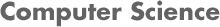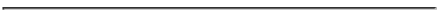### CS534 Artificial Intelligence Homework 4 - Cisco Spring 2013

#### PROF. CAROLINA RUIZ

Due Date: May 7, 2013 at the beginning of class• Instructions:
• Read Chapters/Sections 7.1-7.5, 8, 9.1, 9.2.1-9.2.2, 9.5.1-9.5.3, 9.5.6 of the textbook.
• See multiple examples of forward chaining, backward chaining, and resolution in the solutions to homework and exam problems on the webpages of my offerings of CS4341 (ugrad AI).
• Below, I follow the notation in the book: Capital letters denote constants, and lowercase letters denote variables.
• This is an individual homework. Please state in your homework submission any sources you used in constructing your solutions, as well as whom you discussed these homework problems with.

• Problems: Turn in written solutions to each of the problems below at the beginning of class when the homework is due.

1. Problem 1:

In this problem, we will use 1st order logic to represent and reason with knowledge about the wumpus world.

1. Read Section 8.3.4. It provides a 1st order representation of the wumpus world. In particular, consider the following assertions in our KB:
• (A1). ∀x,y,a,b [Adjacent([x,y],[a,b]) ⇔ [(x=a ∧ (y=b-1 ∨ y=b+1)) ∨ (y=b ∧ (x=a-1 ∨ x=a+1))]]
• (A2). ∀s [Breezy(s) ⇔ ∃ r [Adjacent (r,s) ∧ Pit(r)]]
2. Let's consider again the situation stated in Section 7.2:
• (K1) ¬ Breezy([1,1])
• (K2) ¬ Breezy([1,2])
• (K3) Breezy([2,1])
3. Our agent is trying to determine whether or not there is a pit in cell [3,1]. So, let's express this as:
• (C) Pit([3,1])
4. Let's determine whether C follows from our Knowledge Base A1, A2, K1, K2, and K3 or not. To simplify life, let assume that our inference engine will start by instantiating (that is, generating particular cases of) A2 with all the possible Adjacent relationships expressed in A1 for the cells explicitly mentioned in KD (namely, [1,1], [1,2], [2,1]). For instance, our inference engine will use A1, A2, and K1 to generate:
• (A2.1). Breezy([1,1]) ⇔ [Pit([1,2]) ∨ Pit([2,1])]
Here is what you need to do:
1. (5 points) Construct all possible instantiations of A1 that follow from A2, K1, K2, and K3, as illustrated above.
2. (20 points) Use all those instatiations of A1 together with K1,K2, and K3 to prove C using a proof by refutation using resolution. Follow the process described in Section 7.5.2 step by step. Show your work in detail.
3. (5 points) What can you say about the presence of a pit in location [2,2] at this point? Explain.
4. (5 points) Could you use forward chaining or backward chaining to prove C from the instations of A2 that were generated? Explain your answer.

2. Problem 2:

In this problem, you will show that the following assertions (A1), (A2), (A3), and (A4) together entail (C) by constructing a proof by refulation using resolution.

• (A1). Every edible mushroom or poisonous mushroom is a mushroom.
∀x [Edible(x) ∨ Poisonous(x) ⇒ Mushroom(x)]

• (A2). Every mushroom is grey, white, or yellow.
∀y [Mushroom(y) ⇒ Grey(y) ∨ White(y) ∨ Yellow(y)]

• (A3). No edible mushroom is yellow.
∀z [Edible(z) ⇒ ¬ Yellow(z)]

• (A4). No mushroom that is organic is grey.
∀w [Mushroom(w) ∧ Organic(w) ⇒ ¬ Grey(w)]

• (C). If no mushroom is white, then no edible mushroom is organic.
[∀i [Mushroom(i) ⇒ ¬ White(i)]] ⇒ [¬∃j [Edible (j) ∧ Organic(j)]]
1. Negate the conclusion (C).
2. (25 points) Convert each of the sentences (A1), (A2), (A3), (A4), and ¬ (C) to Conjuctive Normal Form (CNF) following the procedure described in Section 9.5.1 of the textbook step by step. Show your work.
3. (25 points) Apply resolution until you reach the empty clause. At each step, show which two clauses are resolved, and what clause is obtained as a result. Show your work.

3. Problem 3:
• (25 points) Search the web for a real-world AI application that uses mathematical logic for knowledge representation, and logical inference. Describe the inner works of this application in as much detail as possible. Provide links and references to all sources you use. The following suggested references posted on the course webpage: might help you get started.